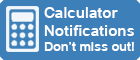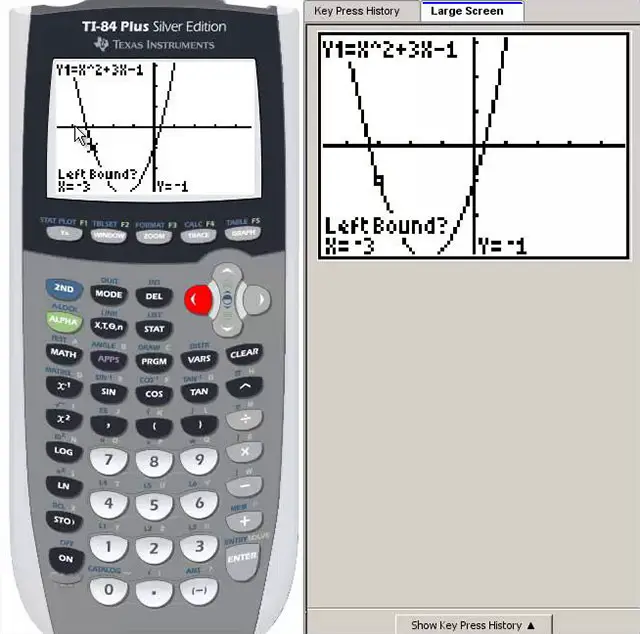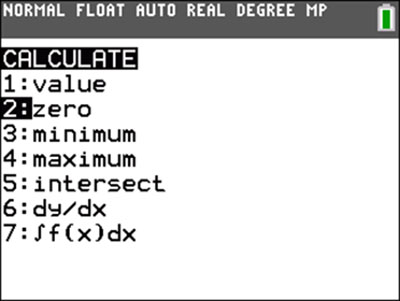Calculator Library
Free Online CalculatorsHome » 87 Calculators » Blog » How To Find Zeros On A Graphing Calculator

Huge Domain Name Sale | Free Domains
Domain names on sale at crazy prices. Register a new domain for FREE when you buy another service.

## HOW TO FIND ZEROS ON A GRAPHING CALCULATORGraphing calculators are essential tools for many math and science courses, allowing students to quickly and easily plot graphs, solve equations and perform many other mathematical tasks. One particularly valuable feature of these calculators is the ability to find a function's zeros, or roots. In this article, we'll cover the steps for finding zeros on a graphing calculator and provide some tips and tricks for getting the most out of this feature.

## What are Zeros and Why are They Important?

Before diving into the details of finding zeros on a graphing calculator, it's essential to understand what zeros are and why they are important.

A function's zero, or root, is a value of the input (x) that results in an output (y) of zero. In other words, it's a point at which the function crosses the x-axis. Zeros are essential because they can give us insight into the behavior of a function. For example, if we know that a function has a zero at x=2, it tells us the function will cross the x-axis at that point.

## Steps For Finding Zeros on a Graphing CalculatorNow that we have a basic understanding of what zeros are and why they are essential, let's go over the steps for finding zeros on a graphing calculator.

### Step 1: Enter the Function

The first step in finding zeros on a graphing calculator is to enter the function for which you want to find the roots. This is typically done by pressing the y= button and then typing in the function using the calculator's keypad. For example, to enter the function y = x^2 - 3x + 2, you would press y=, type "x^2-3x+2" and then press the enter button.

### Step 2: Plot the Graph

Once you've entered the function, the next step is to plot the function's graph. This is typically done by pressing the graph button, which will display the function's graph on the calculator's screen. If the graph isn't displayed correctly, you may need to adjust the viewing window by pressing the window button and entering the desired viewing range for the x- and y-axes.

### Step 3: Find the Zeros

Now that the function's graph is displayed on the screen, the next step is to find the zeros. There are a few different methods for doing this, depending on the type of graphing calculator you're using.

1.Using the "zero" function: Many graphing calculators have a built-in "zero" function that allows you to find the zeros of a function by simply pressing a button. To use this function, you'll typically need to select the function for which you want to find the zeros and then press the zero button or select "zero" from the menu. On a TI-84, this menu is found by pressing 2nd CALC 2. The calculator will then display the zeros of the function on the graph and the x-coordinates of the zeros in the table.
2. Using the intersection of the x-axis: Another method for finding the zeros of a function is to look for the points where the function's graph intersects the x-axis. To do this, you'll need to ensure that the graph's gridlines are turned on, which can typically be done by pressing the "grid" button. Then, simply look for the points where the function's graph crosses the x-axis. The x-coordinates of these points are the zeros of the function.
3. Using the "root" function: If your graphing calculator has a "root" function, you can also find the zeros of a function. To use the "root" function, you'll typically need to enter the function and the range of x-values you want to search for roots. The calculator will then display the function's zeros within the specified range.

## Tips and Tricks

Here are some tips and tricks for finding zeros on a graphing calculator:

• Make sure that the window is set correctly. The window should be set to allow you to see the entire range in which you're searching for zeros. If the window isn't set correctly, you may miss some zeros.
• Use the trace feature to fine-tune your search. The trace feature allows you to move along the function's curve and see the values of x and y at each point. This can be useful for finding zeros that are difficult to see on the graph. To use the trace feature on a TI-84, press the trace button.
• Be aware of extraneous solutions. A common mistake when finding zeros is to include extraneous solutions, which are solutions that may appear to be zeros but are not. For example, if you're looking for zeros of the function y=sqrt(x-4), a zero at x=4 would be an extraneous solution because the function is not defined at that point. To avoid extraneous solutions, check that the function is defined at each zero you find.
• Use the intersect feature to find zeros of two functions. The intersect feature allows you to find the points at which two functions intersect, which can be helpful for finding zeros of the two functions. To use the intersect feature on a TI-84, press the 2nd button followed by the intersect button.
• Use the symmetry of the function to your advantage. Many functions are symmetrical about the x-axis, meaning that if a zero occurs at a given value of x, there will also be a zero at that value's negative. For example, if a function has a zero at x=3, it will also have a zero at x=-3. By taking advantage of this symmetry, you can save time by only searching for zeros in one half of the range.
• Be aware of multiple zeros. Some functions may have more than one zero in a given range. You may need to search multiple times using different ranges to find all of the zeros.
• Use the guess and check method. If you're having trouble finding a zero using the zero feature, try using the guess and check method. This involves guessing a value for x and then evaluating the function to see if the result is zero. You can adjust your guess and try again if the result isn't zero. This method can be time-consuming, but it can be helpful in finding zeros that are difficult to locate using other methods.
• Use the polynomial root finder for polynomial functions. If you're working with a polynomial function (a function of the form y=ax^n+bx^(n-1)+...+zx+k), you can use the polynomial root finder to find all of the zeros quickly. On a TI-84, this feature is accessed by pressing the 2nd button then the zero button.
• Use the "table" function. Some graphing calculators have a "table of values" feature that allows you to see the values of x and y for a range of x-values. This can be a useful tool for finding zeros, as you can easily see the values of x and y at each point and determine when y equals zero.
• If you're having trouble finding the zeros of a function because the graph is too cluttered, you can try turning off some of the features on the graph to make it easier to see. For example, you can turn off the gridlines or the labels on the axes to make the graph less cluttered.
• When finding zeros, it's essential to be aware of the accuracy of the results. Depending on the function and the range of x-values, the calculator may not be able to find zeros with high precision. Make sure to check the accuracy of the results and adjust the range as needed if the results are not accurate enough.
• In addition to finding zeros, you can also use a graphing calculator to find other vital points on a function, such as local extrema (maxima and minima) and inflection points. To find these points, you can use the calculator's features such as the maximum and minimum functions or analyze the function's derivative.

## Other Methods To Find Zeros Of A Function

While finding zeros on a graphing calculator is convenient and efficient, it's not the only way to find zeros of a function. Here are a few other ways that you can find zeros of a function:

• Analytically: One method to find zeros of a function is to solve the equation analytically. This involves using algebraic techniques to find the roots of the equation. This method can be helpful for finding zeros of simple functions, but it becomes increasingly difficult for more complex functions.
• Numerically: Another option is to use a numerical method. This involves using an iterative process to approximate the zeros of the function. There are several numerical methods that can be used to find zeros, including the bisection method, the Newton-Raphson method and the secant method.
• Graphically: A third option is to plot the function and find the points where the graph crosses the x-axis. This method can be helpful for finding zeros of simple functions, but it becomes increasingly difficult for more complex functions.

## Other Applications of Finding Zeros

In addition to analyzing the behavior of a function, finding zeros on a graphing calculator has several other applications. For example, you can use zeros to solve equations with no closed-form solution, such as those involving radicals or logarithms. You can also use zeros to find the points of intersection between two functions, which can be helpful in finding the solution to a system of equations.

Another application of finding zeros on a graphing calculator is optimization. By finding the zeros of the derivative of a function, you can determine the points at which the function has a local maximum or minimum. This can be useful for finding the optimal solution to a problem, such as finding the minimum cost of a production process or the maximum profit of a business.

## Troubleshooting Common Problems

While finding zeros on a graphing calculator is generally a straightforward process, there are a few common problems that you may encounter. Here are some troubleshooting tips for dealing with these problems:

• The calculator doesn't find any zeros:
If the calculator doesn't find any zeros, it could be because there are no zeros within the specified range. It could also be because the function isn't defined within the range or it's discontinuous within the range. Ensure that the function is defined and continuous within the range and adjust the range as needed.
• The calculator finds extraneous solutions:
As mentioned earlier, extraneous solutions appear to be zeros but are not. To avoid extraneous solutions, check that the function is defined at each zero you find.
• The calculator finds complex zeros:
Some functions may have complex zeros, which have both real and imaginary components. If the calculator finds a complex zero, make sure that you've selected the option to display complex solutions. On a TI-84, you can do this by pressing the 2nd button, followed by the format button and selecting the "a+bi" option.

## Real-World Examples

Now that we have gone over the steps and techniques for finding zeros on a graphing calculator let's look at a few examples of how this tool can be used in the real world.

### Example 1: Solving an Equation

Suppose we want to find the value of x that satisfies the equation x^3 - 6x^2 + 11x - 6 = 0. We can use a graphing calculator to find the zeros of this equation.

To do this, we would enter the equation into the calculator and set the window to a suitable range. Then, we would use the zero feature to find the zeros within the specified range. The calculator would then display the values of x at which the zeros occur.

### Example 2: Optimization

Suppose we have a function that represents the cost of producing a particular product as a function of the number of units produced. We want to find the value of x that minimizes the cost.

To do this, we can use the derivative of the cost function to find the points at which the function has a local minimum. We can then use the zero feature on the derivative to find the zeros, the points at which the derivative is zero. The value of x at these points will be the values that minimize the cost function.

### Example 3: Intersection of Two Functions

Suppose we have two functions representing a certain product's supply and demand. We want to find the point at which the two functions intersect, which will represent the equilibrium price.

To do this, we can use the intersect feature on the graphing calculator to find the points at which the two functions intersect. The value of x at these points will be the equilibrium price.

Here are some frequently asked questions about finding zeros on a graphing calculator:

Q: Can I find zeros of non-polynomial functions on a graphing calculator?

A: Yes, you can find zeros of non-polynomial functions on a graphing calculator. While the polynomial root finder only applies to polynomial functions, you can use the zero feature to find zeros of any function.

Q: Can I find all of the zeros of a function on a graphing calculator?

A: It depends on the function. Some functions may have infinite zeros, while others may have a finite number. In general, you can find all of the zeros of a function within a specific range on a graphing calculator. However, you may need to search multiple times using different ranges to find all the zeros.

Q: How do I find the x-intercept of a function on a graphing calculator?

A: The x-intercept of a function is a zero of the function. To find the x-intercept on a graphing calculator, you can use the zero feature to find the function's zeros. The x-intercept will be the value of x at which the zero occurs.

Q: Can I find zeros of a function on a graphing calculator if the function isn't continuous?

A: No, you can't find zeros of a function on a graphing calculator if the function isn't continuous. A graphing calculator's zero feature will only find continuous function zeros. If the function is discontinuous, you must find the zeros manually or use another method.

## Conclusion

In this article, we've seen that finding the zeros of a function using a graphing calculator is a relatively straightforward process that can save you time and effort when solving math and science problems. By following the steps outlined above, you can quickly and easily find a function's roots using various methods, depending on the type of calculator you're using and the complexity of the function.

If your function doesn't have any zeros or has an infinite number of zeros, you can still use advanced techniques like the "intersect" function to find the x-coordinates of the points where the curve of the function comes closest to the x-axis. With a little practice, you'll find the zeros of any function with confidence and ease.

 Previous Post« How Do You Calculate BMI? Next PostHow Do Calculators Work? »